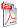Issue archive

https://doi.org/10.15255/KUI.2022.048
Published: Kem. Ind. 72 (3-4) (2023) 169–178
Paper reference number: KUI-48/2022
Paper type: Original scientific paper
Download paper:PDFMulticomponent Adsorption Capacity Forecasting Based on Support Vector Machine with Dragonfly Algorithm

R. Moumen, M. Laidi, S. Hanini, M. Hentabli and A. Ibrir

##### Abstract

The predictability of the adsorption capacity of the multicomponent adsorption system was modelled using Support Vector Machine (SVM). Two SVM models were built and compared. In the first model, the SVM method was used with an already built-in optimisation algorithm. However, in the second model, the SVM method was used by means of a very recent and efficient optimisation algorithm: the Dragonfly Algorithm (DA). The models’ accuracy was evaluated by three well-established statistical metrics (root mean squared error RMSE, determination coefficient R2, and correlation coefficient R). The used data were collected from previous experimental papers published in literature containing all kinds of pollutants, such as heavy metal ions, dyes, and organic compounds, and different natural/synthetic adsorbents. The dataset contained five important variables with 1023 points; the variables were divided into four inputs (molecular weight, equilibrium concentrations of adsorbate, specific area of adsorbent, and temperature), and one output (adsorption capacity at equilibrium). The data were divided using the holdout function into two subsets (80 % for training set, and 20 % for test set). The programming stage was carried out using MATLAB software. The results showed that the optimised DA-SVM model with RBF-Gaussian kernel function had good ability for global search combined with high prediction accuracy, with R2 = 0.997, R = 0.998, and RMSE = 2.539. The obtained model can be used to predict the efficiency of the adsorption system, and provides a tool for process optimisation responding to changes in operating conditions. A new graphical user interface (GUI) was developed with MATLAB GUI to estimate accurately the desired responses by using the best DA-SVM model.

##### Keywords

multicomponent adsorption, MATLAB GUI, pollutants, support vector regression, Dragonfly algorithm